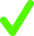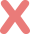Angle 1  =  °
Angle 2  =  °
Angle 3  =  °
Angle 4  =  °
Angle 5  =  °
Angle 6  =  °
Angle 7  =  °
Angle 8  =  °
00ix

Drag any blue point to change the angles where the transversal crosses the parallel lines.